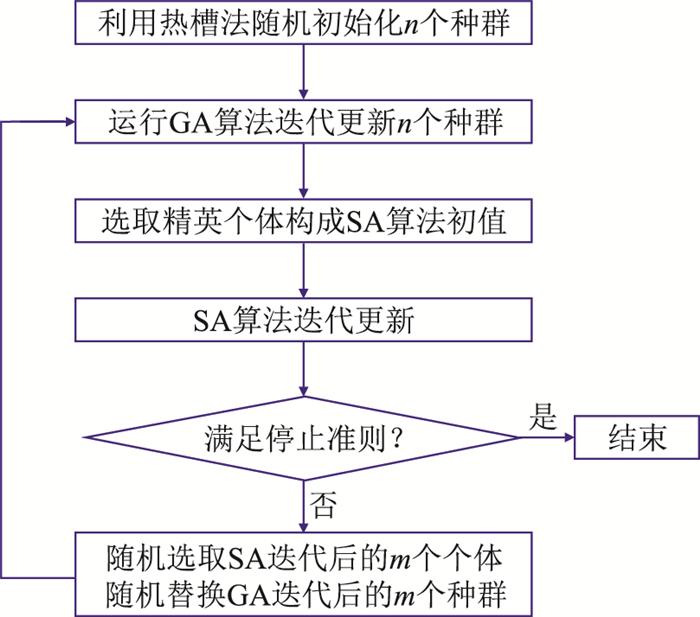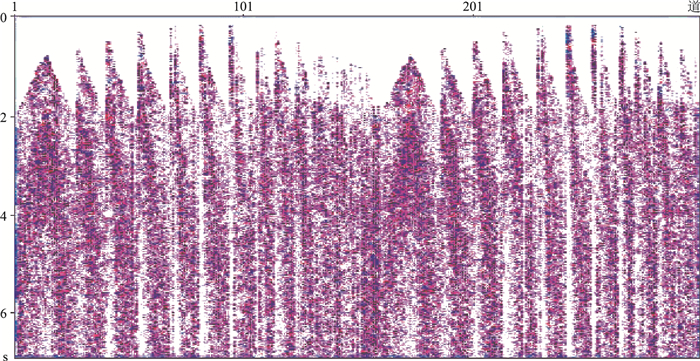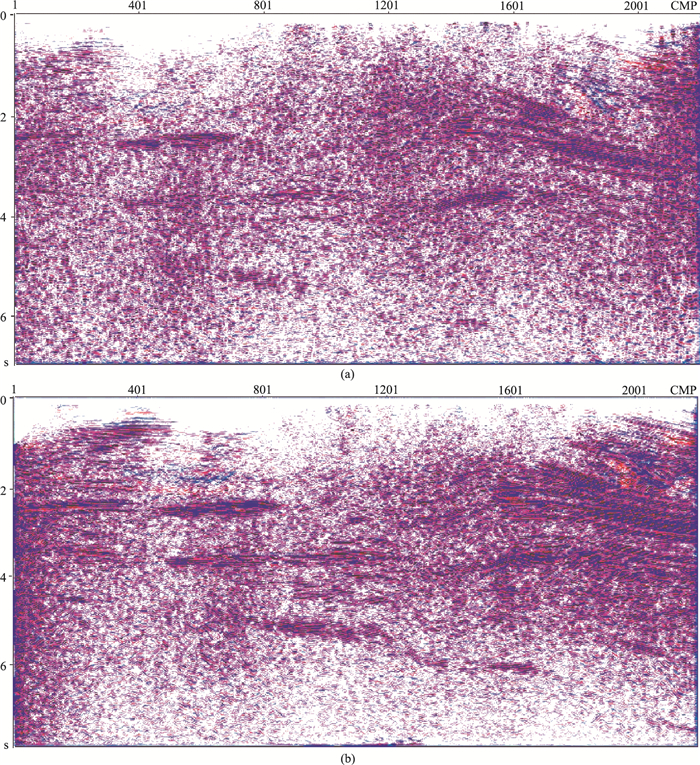﻿ 遗传和模拟退火混合寻优一步法三维CRS叠加
 石油地球物理勘探2021, Vol. 56Issue (2): 283-288  DOI: 10.13810/j.cnki.issn.1000-7210.2021.02.0090文章快速检索 高级检索

### 引用本文SUN Xiaodong, HOU Mengrui, REN Lijuan, WANG Weiqi, LI Zhenchun. An improved one-step 3D CRS stacking method based on hybrid optimization algorithm. Oil Geophysical Prospecting, 2021, 56(2): 283-288. DOI: 10.13810/j.cnki.issn.1000-7210.2021.02.009.### 作者简介侯孟睿, 山东省青岛市黄岛区长江西路66号中国石油大学(华东)地球科学与技术学院, 266580。Email: 569096595@qq.com

### 文章历史

① 中国石油大学(华东)深层油气重点实验室, 山东青岛 266580;
② 中国石油大学(华东)山东省油藏地质重点实验室, 山东青岛 266580;
③ 中海石油(中国)有限公司湛江分公司南海西部石油研究院, 广东湛江 524000

An improved one-step 3D CRS stacking method based on hybrid optimization algorithm
SUN Xiaodong①② , HOU Mengrui, REN Lijuan , WANG Weiqi , LI Zhenchun
① Key Laboratory of Deep Oil & gas, China University of Petroleum(East China), Qingdao, Shandong 266580, China;
② Shandong Provincial Key Laboratory of Reservoir Geology, China University of Petroleum(East China), Qingdao, Shandong 266580, China;
③ Nanhai Western Petroleum Research Institute, Zhanjiang Branch, CNOOC, Zhanjiang, Guangdong 524000, China
Abstract: Common reflection surface (CRS) stacking makes full use of seismic data within the range of a Fresnel radius, and maximizes the signal-to-noise ratio without reducing the resolution. It is a po-werful imaging means of the seismic data with low signal-to-noise ratio. At the same time, CRS stacking takes into account the inclination and local curvature of the underground reflector, so the imaging accuracy is higher. In conventional three-dimensional CRS stacking, the accuracy of the eight parameters obtained sequentially in multiple steps is low, and affects the final stacking effect. We propose a one-step three-dimensional CRS stacking strategy which combines the rapid search of genetic algorithm and the global convergence of simulated annealing algorithm. It uses a multi-group hierarchical hybrid parallel optimization algorithm that mixes the two algorithms, that is, a thermal slot method is used to generate initial population in the top layer, the middle layer executes a parallel genetic iterative algorithm to achieve population evolution, and the bottom layer uses a simulated annealing algorithm to achieve global optimization, and the genetic and simulated annealing hybrid algorithm is used for hierarchical parallel computing. The CRS stacking method and the design of the hybrid algorithm significantly improve the optimization cost and accuracy of parameters. Tests on real data have verified the practicability of the one-step 3D CRS stacking method.
Keywords: common reflection surface stack    parameter coupling    genetic algorithm    simulated annea-ling    parameter optimization
0 引言

CRS叠加技术最早由德国的Hurbal提出，是一种与宏观速度模型无关的地震成像方法。用于描述CRS时距公式的算子有双曲CRS算子、隐式CRS算子、非双曲CRS算子和多聚焦算子等。这些算子在速度横向变化较小时相差不大，但在速度横向变化剧烈时存在一定的差异。CRS叠加的理论基础是几何地震学，考虑了反射层的局部特征和第一菲涅耳带内的全部反射。CRS叠加引入反射段及反射面理论，在几何地震学基础上研究第一菲涅耳带范围内的反射，叠加次数得到提高，在提高信噪比的同时满足高分辨率的成像要求，是低信噪比、低覆盖次数地震资料的主要处理手段[3-7]

1 三维CRS叠加算子及波场参数

 \begin{aligned} &t_{\mathrm{hyp}}^{2}\left(\boldsymbol{m}_{\mathrm{D}}, \boldsymbol{h}\right)=\left[t_{0}\left(\boldsymbol{m}_{0}, 0\right)+\frac{2 \sin \alpha}{v_{0}} \boldsymbol{\varPhi} \boldsymbol{m}_{\mathrm{D}}\right]^{2}+\\ &\frac{2 t_{0}\left(\boldsymbol{m}_{0}, 0\right)}{v_{0}} \times\left(\boldsymbol{m}_{\mathrm{D}}^{\mathrm{T}} \boldsymbol{\varTheta}^{\mathrm{T}} \boldsymbol{\varPhi}^{\mathrm{T}} \boldsymbol{K}_{\mathrm{N}} \boldsymbol{\varPhi} \boldsymbol{\varTheta} \boldsymbol{m}_{\mathrm{D}}+\right.\\ &\left.\boldsymbol{h}^{\mathrm{T}} \boldsymbol{\varTheta}^{\mathrm{T}} \boldsymbol{\varPhi}^{\mathrm{T}} \boldsymbol{K}_{\mathrm{NIP}} \boldsymbol{\varPhi} \boldsymbol{\varTheta} \boldsymbol{h}\right) \end{aligned} (1)

 $\boldsymbol{W}_{\mathrm{Z}}=\frac{2}{v_{0}} \sin \alpha \boldsymbol{\varPhi}$ (2)
 $\boldsymbol{N}_{\mathrm{H}}=\frac{2 t_{0}\left(\boldsymbol{m}_{0}, 0\right)}{v_{0}} \boldsymbol{\varTheta}^{\mathrm{T}} \boldsymbol{\varPhi}^{\mathrm{T}} \boldsymbol{K}_{\mathrm{N}} \boldsymbol{\varPhi} \boldsymbol{\varTheta}$ (3)
 $\boldsymbol{M}_{\mathrm{H}}=\frac{2 t_{0}\left(\boldsymbol{m}_{0}, 0\right)}{v_{0}} \boldsymbol{\varTheta}^{\mathrm{T}} \boldsymbol{\varPhi}^{\mathrm{T}} \boldsymbol{K}_{\mathrm{NIP}} \boldsymbol{\varPhi} \boldsymbol{\varTheta}$ (4)

 \begin{aligned} t_{\text {hyp }}^{2}\left(\boldsymbol{m}_{\mathrm{D}}, \boldsymbol{h}\right)=&\left[t_{0}\left(\boldsymbol{m}_{0}, 0\right)+\boldsymbol{W}_{\mathrm{Z}} \boldsymbol{m}_{\mathrm{D}}\right]^{2}+\\ &\boldsymbol{m}_{\mathrm{D}}^{\mathrm{T}} \boldsymbol{N}_{\mathrm{H}} \boldsymbol{m}_{\mathrm{D}}+\boldsymbol{h}^{\mathrm{T}} \boldsymbol{M}_{\mathrm{H}} \boldsymbol{h} \end{aligned} (5)
2 三步法三维CRS叠加实现策略

(1) 仅考虑CMP道集，即令mD=0，式(5)可写为

 $t_{\mathrm{hyp}}^{2}(\boldsymbol{h})=t_{0}^{2}\left(\boldsymbol{m}_{0}, 0\right)+\boldsymbol{h}^{\mathrm{T}} \boldsymbol{M}_{\mathrm{H}} \boldsymbol{h}$ (6)

(2) 设KN=0，并且在零炮检距数据体中设h=0，由式(5)可得

 $t_{\mathrm{hyp}}\left(\boldsymbol{m}_{\mathrm{D}}\right)=t_{0}\left(\boldsymbol{m}_{0}, 0\right)+\boldsymbol{W}_{\mathrm{Z}} \boldsymbol{m}_{\mathrm{D}}$ (7)

(3) 在零炮检距情况下，即h=0时，式(5)可写为

 $t_{\mathrm{hyp}}^{2}\left(\boldsymbol{m}_{\mathrm{D}}\right)=\left[t_{0}\left(\boldsymbol{m}_{0}, 0\right)+\boldsymbol{W}_{\mathrm{Z}} \boldsymbol{m}_{\mathrm{D}}\right]^{2}+\boldsymbol{m}_{\mathrm{D}}^{\mathrm{T}} \boldsymbol{N}_{\mathrm{H}} \boldsymbol{m}_{\mathrm{D}}$ (8)

3 一步法实现三维CRS叠加

GA算法和SA算法都属于群体智能型全局寻优算法，在面对小规模的优化问题时，不管是参数优化效率还是参数求解质量方面，都有着成功的表现。但是随着地震勘探转向更复杂的区域，实际地震资料处理也逐渐由二维扩展到三维，需要处理的地震数据体越来越大，单纯的GA算法或SA算法在面对三维CRS叠加中八参数的求解问题时，无论是参数求解的效率还是精度均不理想[6, 8-13]

GA算法在算法运行初期，收敛速度很快，可以在短时间内收敛到局部最优解，但随着算法中种群的进化，算法的收敛速度开始变缓，无法精确地收敛到全局最优解，即出现了“早熟收敛”现象。这也印证了GA算法简单易实现，但搜索精度不高，且算法得出的解并不一定是全局最优的特点。而且标准的GA算法是随机产生初始群体，没有约束条件，可能出现：一是初始群体中会产生一些远离最优解的个体，导致求解用时长、精度低；二是初始群体的多样性过低，容易收敛到局部极小值。而在三维CRS叠加中八参数寻优过程中，每个参数的精度都非常重要，直接影响叠加的效果。SA算法虽然收敛速度缓慢，但在理论上已经证明其搜索过程是不断接近最优解直至以概率1最终收敛于全局最优解。SA法在处理复杂且庞大的全局寻优问题时，前期的求解速度十分缓慢，因此在面对复杂的三维地震数据体时显得更加乏力。

GA算法具有快速寻优能力，SA算法有很好的全局收敛特性，两者有着几乎互补的优势。本文结合分层混合的思想，根据全局优化算法的并行性及二者的算法特点，提出GA与SA混合的多种群精英分层式混合并行寻优(简称GA-SAHP)算法，其种群个体组织方式从上到下总体可以分为三层：

(1) 顶层是根据SA算法中的热槽法，通过概率分布函数随机产生三维CRS叠加中八个相关参数的若干个候选值，这些值随机分配到中层n个GA算法中形成的每个种群的初始状态，形成初始种群；

(2) 中层则是n个独立运行的遗传迭代过程，可以在一定程度上保证解的多样性，每个种群独立交叉、变异、选择形成新解，这些局部最优解将组成精英种群，为算法底层SA算法提供初始值；

(3) 底层采用SA算法对中层选出的精英种群进行迭代，对全局最优解进行搜索，迭代一定次数之后，判断是否满足SA算法中的外循环停止准则，若满足则输出全局最优解；否则，中层的GA算法在SA算法产生的解中随机抽取一定数量的解放入中层的种群中继续进化，直至得出全局最优解，输出相干值最大的八个参数。图 1 GA-SAHP算法的流程

4 实际地震资料处理

CRS叠加适用于低信噪比、低覆盖次数地震数据的处理。为比较常规CRS叠加与基于GA-SAHP算法搜索参数的一步法CRS叠加效果，对实际海上三维地震数据进行CRS叠加处理。图 2 实际低信噪比地震数据的CMP道集图 3 常规(a)与基于GA-SAHP算法(b)的CRS叠加剖面对比

5 结束语

(1) 改进后的CRS叠加，对低信噪比的深层成像效果更好，中深层的反射同相轴连续性增强。

(2) 在参数搜索效率方面，基于GA-SAHP算法参数搜索的一步法高于常规三步法。

  Hubral P. Computing true amplitude reflections in a laterally inhomogeneous earth[J]. Geophysics, 1983, 48(8): 1051-1062. DOI:10.1190/1.1441528  Jäger R, Mann J, H cht G, et al. Common-reflection-surface stack: Image and attributes[J]. Geophysics, 2001, 66(1): 97-109. DOI:10.1190/1.1444927  Barros T, Ferrari R, Krummenauer R, et al. Differential evolution based optimization procedure for automatic estimation of the common reflection surface traveltime parameters[J]. Geophysics, 2015, 80(6): WD189-WD200. DOI:10.1190/geo2015-0032.1  Bruno P. High-resolution seismic imaging in complex environments: A comparison among common-reflection-surface stack, common-midpoint stack, and pre-stack depth migration at the Ilva-Bagnoli brownfield site[J]. Geophysics, 2015, 80(6): B203-B214. DOI:10.1190/geo2014-0488.1  陈宝书, 杨锴, 汪小将. 基于两步弥散算子的共反射面元射线束成像方法研究[J]. 地球物理学报, 2020, 63(1): 270-286. CHEN Baoshu, YANG Kai, WANG Xiaojiang, et al. The CRS-BEAM-PSDM based on two-step-smearing operator[J]. Chinese Journal of Geophysics, 2020, 63(1): 270-286.  杨锴, 马在田, 罗卫东. 输出道成像方式的共反射面元叠加方法Ⅱ——实践[J]. 地球物理学报, 2006, 49(3): 895-902. YANG Kai, MA Zaitian, LUO Weidong. Common reflection surface stack by the outplan, Ⅱ: Practice[J]. Chinese Journal of Geophysics, 2006, 49(3): 895-902. DOI:10.3321/j.issn:0001-5733.2006.03.035  王华忠, 杨锴, 马在田. 共反射面元叠加的应用理论——从共反射点到共反射面元[J]. 地球物理学报, 2004, 47(1): 137-142. WANG Huazhong, YANG Kai, MA Zaitian. An applied theory on common reflection surface stack: from common reflection point to common reflection surface[J]. Chinese Journal of Geophysics, 2004, 47(1): 137-142. DOI:10.3321/j.issn:0001-5733.2004.01.021  Garabito G. Global optimization strategies for implementing 3D common reflection surface stack using the very fast simulated annealing algorithm: Application to real land data[J]. Geophysics, 2018, 83(4): V253-V261. DOI:10.1190/geo2017-0836.1  Xie Y J, Gajewski D. 3D wavefront attribute determination and conflicting dip processing[J]. Geophysics, 2018, 83(6): V325-V343. DOI:10.1190/geo2017-0792.1  Rad P, Schwarz B, Gajewski D, et al. Common reflection surface based prestack diffraction separation and imaging[J]. Geophysics, 2018, 83(1): S47-S55. DOI:10.1190/geo2016-0445.1  倪瑶, 杨锴. 基于GPU计算平台实现三维输出道方式的共反射面元(3D-CRS-OIS)叠加[J]. 石油地球物理勘探, 2013, 48(1): 49-57. NI Yao, YANG Kai. 3D common reflection surface stack algorithm based on GPU with the output imaging scheme[J]. Oil Geophysical Prospecting, 2013, 48(1): 49-57.  Dell S, Gajewski D. Common-reflection-surface-based workflow for diffraction imaging[J]. Geophysics, 2011, 76(5): S187-S195. DOI:10.1190/geo2010-0229.1  Bergler S, Hubral P, Marchetti P, et al. 3D common reflection surface stack and kinematic wavefield attri-butes[J]. The Leading Edge, 2002, 21(10): 1010-1015. DOI:10.1190/1.1518438  Duveneck E. Velocity model estimation with data-derived wavefront attributes[J]. Geophysics, 2004, 69(1): 265-274. DOI:10.1190/1.1649394  Martina G, Sergius D. Velocity-estimation improvements and migration/demigration using the common-reflection surface with continuing deconvolution in the time domain[J]. Geophysics, 2019, 84(4): S229-S238. DOI:10.1190/geo2018-0173.1  Marcelo J. Velocity inversion by global optimization using finite-offset common-reflection-surface stacking applied to synthetic and Tacutu Basin seismic data[J]. Geophysics, 2019, 84(2): R165-R174. DOI:10.1190/geo2017-0117.1  Yin J H, Nakata N. Diffraction imaging using geometric-mean reverse time migration and common-reflection surface[J]. Geophysics, 2019, 84(4): S355-S364. DOI:10.1190/geo2018-0455.1  孙小东, 贾延睿, 李振春, 等. 粒子群算法参数搜索的共反射面元叠加[J]. 石油地球物理勘探, 2019, 54(4): 782-786. SUN Xiaodong, JIA Yanrui, LI Zhenchun, et al. Common reflection surface stack based on parameter searching by PSO[J]. Oil Geophysical Prospecting, 2019, 54(4): 782-786.  陈汉明, 周辉, 田玉昆. 分数阶拉普拉斯算子黏滞声波方程的最小二乘逆时偏移[J]. 石油地球物理勘探, 2020, 55(3): 616-626. CHEN Hanming, ZHOU Hui, TIAN Yukun. Seismic diffraction imaging by reverse time migration in dip angle domain[J]. Oil Geophysical Prospecting, 2020, 55(3): 616-626.  Bauer A, Schwarz B, Gajewski D. Utilizing diffractions in wavefront tomography[J]. Geophysics, 2017, 82(2): R65-R73. DOI:10.1190/geo2016-0396.1  Walda J, Gajewski D. Determination of wavefront attri-butes by differential evolution in the presence of confli-cting dips[J]. Geophysics, 2017, 82(4): V229-V239. DOI:10.1190/geo2016-0346.1  王征, 杨锴, 董水利, 等. 应用叠前时间偏移/反偏移与CRS-OIS叠加削弱倾角歧视影响[J]. 石油地球物理勘探, 2015, 50(5): 839-847. WANG Zheng, YANG Kai, DONG Shuili, et al. Dip discrimination reduction with CRS-OIS[J]. Oil Geophysical Prospecting, 2015, 50(5): 839-847.